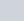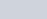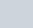Find the angle subtended at the origin by the line segment
Question:

Find the angle subtended at the origin by the line segment whose end points are (0, 100) and (10, 0).

Solution:

The distance $d$ between two points $\left(x_{1}, y_{1}\right)$ and $\left(x_{2}, y_{2}\right)$ is given by the formula

$d=\sqrt{\left(x_{1}-x_{2}\right)^{2}+\left(y_{1}-y_{2}\right)^{2}}$

In a right angled triangle the angle opposite the hypotenuse subtends an angle of.

Here let the given points be A(0,100), B(10,0). Let the origin be denoted by O(0,0).

Let us find the distance between all the pairs of points

$A B=\sqrt{(0-10)^{2}+(100-0)^{2}}$

$=\sqrt{(-10)^{2}+(100)^{2}}$

$=\sqrt{100+10000}$

$A B=\sqrt{10100}$

\begin{aligned} A O &=\sqrt{(0-0)^{2}+(100-0)^{2}} \\ &=\sqrt{(0)^{2}+(100)^{2}} \end{aligned}

$=\sqrt{(0)^{2}+(100)^{2}}$

$A O=\sqrt{10000}$

$B O=\sqrt{(10-0)^{2}+(0-0)^{2}}$

$=\sqrt{(10)^{2}+(0)^{2}}$

$B O=\sqrt{100}$

Here we can see that $A B^{2}=A O^{2}+B O^{2}$.

So,is a right angled triangle with ‘AB’ being the hypotenuse. So the angle opposite it has to be. This angle is nothing but the angle subtended by the line segment ‘AB’ at the origin.

Hence the angle subtended at the origin by the given line segment is.